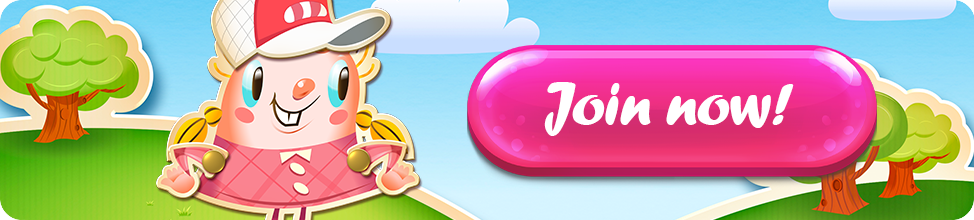Level 1000 Level 2000 Level 3000 Level 4000 Level 5000 Level 6000 Level 7000 Level 8000 Level 9000 Level 10 000 Level 11 000

👻 (ENDED)..MATHS LESSON GUYS..(ENDED) 😟👻

• 110

·
• The answer is : 102

·
• Assume that the skulls are x, the bubblegum is y and the sugar coat is z.

3x=60, so x=20

20+2y=30, so y=5

5-z=3, so z=2

z+x*y=2+(20*5)

·
• The answer is 102 using the PEMDAS method.

·
• =110

·
• edited November 2020

3S=20

S+2P=30

2P=10

P=5

P-C=3

5=C=3

C=2

C+S*P=?

2+(20*5)=?

100+2=?

C+S*P=102

(S=Sour Skull, P-Bubblegum Pop, C=Sugar Coat

·

·
• I would love to know how some answers were calculated🤣

·
• 💀 = 20 (20*3 = 60)

🍭 = 5 (20 + 2*5 = 30)

❄️🍭 = 2 (5 - 3 = 2)

❄️🍭 + 🍭*💀 = 2 + 5*20 = 102

102 it is :)

·
• Thank you for the contest.🤗

Thank you for the tag @DiamondLim

·
• Hello @KimElston and @Elsa!

Another fun math contest! This is my answer: 2 + 20 = 22 then 22 x 5 = 110. My answer is 110.

·